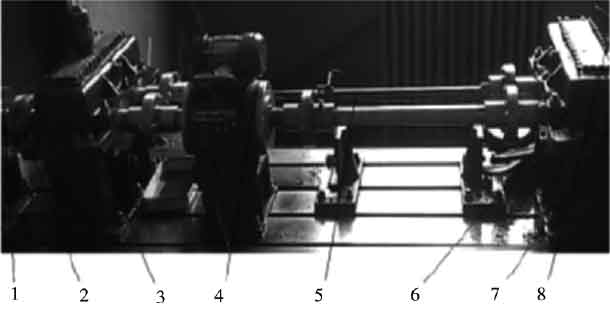# Fatigue test of gearbox bearing based on maximum kurtosis entropy deconvolution of lpso1.Adjustable speed motor 2. Coupling 3. Gearbox 4. Speed change instrument 5. Torsion bar 6. Test gearbox 7. Acceleration sensor 1 8. Acceleration sensor 2

In order to verify the superiority of this method in engineering application, relevant tests are carried out on the closed power flow test bench. The main components of the test bench include the test gearbox, console, motor, three-way acceleration sensor, etc. the motor power is 30 kW, and the speed range is 120 RGMin ~ 1300 R / min. the specific test platform is shown in Figure 1. In order to verify the effectiveness of the method, the defective gear and the cracked bearing outer ring are tested. The specific fault forms are shown in Fig. 2 and Fig. 3, and the accelerometer collecting vibration signal is yd77sa. The sensitivity is 0.01 V / MS2, the bearing model is 3222, the sampling frequency is 8000 Hz, and the number of teeth is 18. After simple calculation, the gear meshing frequency is 360hz, and the bearing outer ring failure frequency is 160hz.

Figure 4 is the time domain diagram and frequency domain diagram of the vibration signal collected by the sensor. It can be seen from the frequency domain diagram that there are obvious peaks at 360 Hz and 720 Hz, corresponding to two gear failure frequencies, and the bearing outer ring failure frequency of 160 Hz is submerged by noise.

EEMD is used to decompose the fault signal, and the results are shown in Fig. 5 and Fig. 6. Fig. 5 is a decomposed time domain diagram, and Fig. 6 is a spectrum diagram obtained by spectral transformation. As can be seen from the figure, the signal is adaptively decomposed into 11 layers. The first layer is the high frequency noise component, which does not contain fault information. The second layer has obvious amplitude at frequency 720, which is twice the frequency of gear failure. The third layer, like the second layer, has obvious amplitude at frequency 720, which is twice the frequency of gear failure. The fourth layer has obvious amplitude at frequency 360, i.e. gear failure frequency. Therefore, the second layer, the third layer and the fourth layer are the fault parts of the gear. The fifth layer has obvious amplitude at 160 Hz, which is the characteristic frequency of bearing outer ring fault. Layers 6 to 11 contain no fault information and are pseudo components.

According to the EEMD decomposition results of vibration signals, the high-frequency noise component imf1 is firstly removed, and then the components with mode mixing are reconstructed to eliminate the mode mixing phenomenon. In other words, the components imf2, imf3 and imf4 are reconstructed into CMF1, and imf5 is reconstructed into cmf2. Finally, the pseudo components imf6 ~ imf11 without fault information are deleted. The results of mode reconstruction are shown in Fig. 7. Fig. 7a shows the time domain analysis of the reconstructed component. Fig. 7b shows the envelope frequency domain analysis of the reconstructed component. The fault frequencies of 360 Hz and 160 Hz can be found in the envelope spectrum, but the spectral lines are messy, which is easy to cause misjudgment.